Courses

# Introduction: The Force Method of Analysis - 2 Civil Engineering (CE) Notes | EduRev

## Civil Engineering (CE) : Introduction: The Force Method of Analysis - 2 Civil Engineering (CE) Notes | EduRev

The document Introduction: The Force Method of Analysis - 2 Civil Engineering (CE) Notes | EduRev is a part of the Civil Engineering (CE) Course Structural Analysis.
All you need of Civil Engineering (CE) at this link: Civil Engineering (CE)

Solution with RB as the redundant

2) After selecting  Ras redundant, express all other reactions in terms of the redundant R. This can be accomplished with the help of equilibrium equations.

Thus,

RA = wL − RB                        (7.1a)

and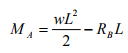(7.1b)

3) Now release the restraint corresponding to redundant reaction RB . Releasing restraint in the present case amounts to removing the support at B. Now on the resulting cantilever beam (please note that the released structure is statically determinate structure), apply uniformly distributed load w and the redundant reaction RB as as shown in Fig. 7.2b. The released structure with the external loads is also sometimes referred as the primary structure.

4) The deflection at B of the released structure (cantilever beam, in the present case) due to uniformly distributed load and due to redundant reaction RB could be easily computed from any one of the known methods (moment area method or unit load method). However it is easier to compute deflection at B due to uniformly distributed load and RB in two steps. First, consider only uniformly distributed load and evaluate deflection at B, which is denoted by (ΔB)1 as shown in Fig. 7.2c. Since RB is redundant, calculate the deflection at B due to unit load at B acting in the direction of RB and and is denoted by (ΔB)as shown in

In the present case the positive direction of redundant and deflections are assumed to act upwards. For the present case, (ΔB)1 and (ΔB)2 are given by,(7.2a)

and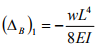(7.2b)

From the principle of superposition, the deflection at B, (ΔB), is the sum of deflection due to uniformly distributed load (ΔB)1 and deflection RBB)2 due to redundant RB . Hence,

ΔB =  (ΔB)1 + RBB)2                                (7.2c)

5) It is observed that, in the original structure, the deflection at B is zero. Hence the compatibility equation can be written as,

ΔB =  (ΔB)1 + RBB)2   = 0                                  (7.3a)

Solving the above equation, the redundant Rcan be evaluated as,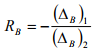(7.3b)

Substituting values of (ΔB)1 and (ΔB)2 , the value of Ris obtained as,

$$R_B = {3wL\over8}$$                             (7.3d)

The displacement at B due to unit load acting at B in the direction of RB is known as the flexibility coefficient and is denoted in this course by aBB.

6) Once Ris evaluated, other reaction components can be easily determined from equations of statics. Thus,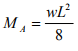(7.4a)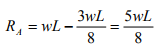(7.4b)

7) Once the reaction components are determined, the bending moment and shear force at any cross section of the beam can be easily evaluated from equations of static equilibrium. For the present case, the bending moment and shear force diagram are shown in Fig. 7.2e.

Solution with MA as redundant

1) As stated earlier, in the force method the choice of redundant is arbitrary. Hence, in the above problem instead of RB one could choose MA as the redundant reaction. In this section the above problem is solved by taking MA as redundant reaction.

2) Now release (remove) the restraint corresponding to redundant reaction MA. This can be done by replacing the fixed support at A by a pin. While releasing the structure, care must be taken to see that the released structure is stable and statically determinate.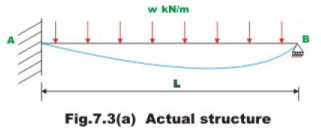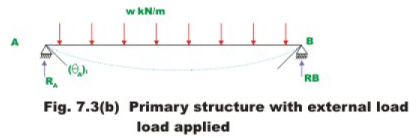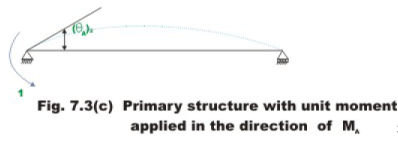3) Calculate the slope at A due to external loading and redundant moment MA. This is done in two steps as shown in Fig. 7.3b and Fig.7.3c. First consider only uniformly distributed load (see Fig. 7.3b) and compute slope at A, i.e.(θA)1 from force displacement relations. Since MA is redundant, calculate the slope at A due to unit moment acting at A in the direction of MA which is denoted by (θA)2 as in Fig. 7.3c. Taking anticlockwise moment and anticlockwise rotations as positive, the slope at A, due to two different cases may be written as,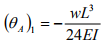(7.5a)

A)2 = L/3EI                           (7.5b)

From the principle of superposition, the slope at A, θA is the sum of slopes (θA)1 due to external load and MAA)2 due to redundant moment MA. Hence

MA = (θA)1 + MAA)2               (7.5c)

4) From the geometry of the original structure, it is seen that the slope at A is zero. Hence the required compatibility equation or geometric condition may be written as,

A) = (θA)1 + MAA)2 = 0                      (7.5d)

Solving fo MA,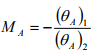(7.5e)

Substituting the values of (θA)1 , and (θA)2 in in equation (7.5e), the value of MA is calculated as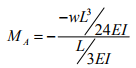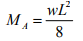(7.5f)

5) Now other reaction components can be evaluated using equilibrium equations. Thus,

RA = 5wL/8                         (7.6a)

RB = 3wL/8                          (7.6b)

Offer running on EduRev: Apply code STAYHOME200 to get INR 200 off on our premium plan EduRev Infinity!

## Structural Analysis

30 videos|122 docs|28 tests

,

,

,

,

,

,

,

,

,

,

,

,

,

,

,

,

,

,

,

,

,

;